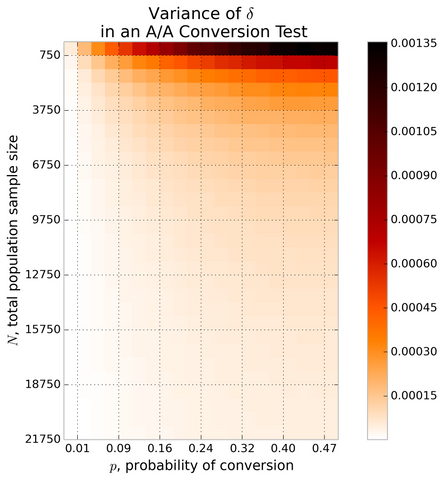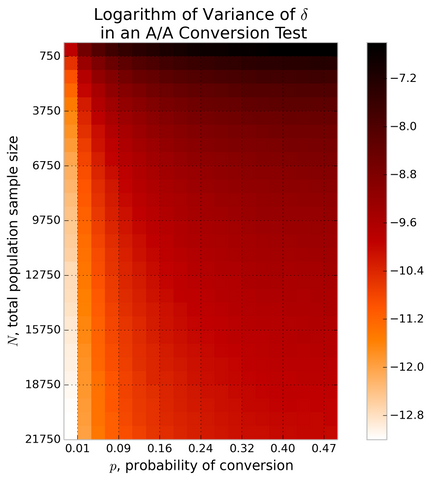# The Class Imbalance Problem in A/B Testing

Posted by Cameron Davidson-Pilon on

## Introduction

If you have been following this blog, you'll know that I employ Bayesian A/B testing for conversion tests (and see this screencast to see how it works). One of the strongest reasons for this is the interpretability of the analogous "p-value", which I call Confidence, defined as the probability the conversion rate of A is greater than B,

$$\text{confidence} = P( C_A > C_B )$$

Really, this is what the experimenter wants - an answer to: "what are the chances I am wrong?". So if I saw a confidence of 1.0 (or as I report it, 100%), I would have to conclude that I know for certain which group is superior.

In practice, I've encountered a 100% confidence a few times, most often in two situations: when my sample size is very low, and when my sample size is very high. The latter situation makes sense: I have lots of data, enough so my testing algorithm can confidently conclude a winner. The former, when I have a small sample size, makes less sense - at first. Suppose the difference between the groups is small, then it doesn't make sense for my algorithm to be so certain in a winner. Instead what is happening is a break down between theory and practice.

## Assumptions of A/B Testing

Let's go back to the beginning. What are the assumptions of A/B testing. Luckily, they are quite short:

1. Before any treatment, your groups are the same.

That's it! The A/B testing algorithm proceeds by applying a treatment to one of the groups and you measure the results in both groups. In practice however, our groups are not the same. The best we can do is have them "statistically" equal, that is to say, equal on average. In practice, we need to rely on the Law of Large Numbers to make our groups statistically equal. Unfortunately, the LLN works for large sample sizes only - you can't assume equal, or even similar, populations with small sample sizes.

## The Class Imbalance Problem

With small sample sizes, and even with medium sample sizes, your groups will be different and there is nothing you can do about it. Perhaps just by chance, more people who were going to convert were put in Bucket A, and thus the treatment's effect is dwarfed by this chance event. This is the class imbalance problem. This is why I was seeing 100% confidence with small sample sizes: by chance a bunch of converters were put into a single bucket and my A/B test algorithm was assuming equal populations.

Let's make this more formal. Define delta, denoted $$\delta$$ as the observed difference between the two groups. $$\delta$$ can be broken down into two parts:

$$\delta = \delta_{ci} + \delta_{T}$$

where $$\delta_{T}$$ is the difference in conversion rates due to the treatment, and $$\delta_{ci}$$ is the difference due to the class imbalance problem. I'm curious about the variance in the observed $$\delta$$, that is, how extreme might my observed $$\delta$$ be. Taking the variance of both sides:

$$\text{Var}(\delta) = \text{Var}(\delta_{ci}) + \text{Var}(\delta_{T})$$

(As the two terms on the right hand side are independent, there will be no correlation term).

### What is the Influence of Class Imbalance?

Let's do an A/A test: that is, let's not apply a treatment to either group. Then $$\delta_{T}=0$$. So any variance we see in the observed delta is due purely to variance from the class imbalance problem. Hence in an A/A test:

$$\text{Var}(\delta) = \text{Var}(\delta_{ci})$$

To find the variance, we'll perform some Monte Carlo simulations of a typical A/A test. Here are the steps:

1. Gather $$N$$ individuals, each who will convert with probability $$p$$.
2. Assign each individual to group $$A$$ with probability .5 (note this does imply exactly half will be in one group, and the other half in the other group).
3. Allow the individuals to convert or not. Compute the observed $$\delta$$ equal to the difference between the observed fraction of conversions to population size.
4. Do the above thousands of times, and compute the variance of the $$\delta$$'s.
5. Do the above again, varying $$N$$ and $$p$$ .

Below is the Python code for this simulation:

Below is a grid of the observed variances, varying $$p$$ and $$N$$:White is good: it implies the LLN has taken over and there is little variance in the observed $$\delta$$. This is where an A/B experiment works: observed difference in the groups are highly likely to be the result of the treatment. If this is hard to read due to the lack of contrast, below is the log-plot of the above:## What Does All This Mean?

It means you have to distrust A/B results will small sample sizes, at least if the variance of the class imbalance problem is of the same order of magnitude as the variance of the treatment effect.

Furthermore, this is another reason why we see these huge "unicorn" experiments (ugh, this is term coined by a recent article that claimed 1000% improvement from an experiment). Suppose there is a positive increase from treatment and that group was assigned significantly more converters just by chance (and the sample size is small enough to allow this), then your estimate of "lift" is going to be much larger than it actually is. Compound this with mistaking the binary problem with the continuous problem, and you've got the recipe for the ﻿1000% improvement fallacy.

## Conclusion

Hopefully, you've learned from my mistake: don't believe anything with small sample sizes, especially if your treatment effect is very small. You can use the above figures to determine the variance at different population sizes and conversion probabilities (how do you know the conversion probability? Well, you don't - best to estimate it with the total conversion rate: total converts / total population). If the variance at that point is too high, then likely your observed delta is being influenced strongly by the class imbalance problem and not the treatment effect. You can find a csv of the data in the gist here.

Thanks to Chris Harland and Putra Manggala for their time discussing these ideas.

## Latest Data Science screencasts available

• I think there are at least a few more assumptions you make in your testing that have gone unsaid. First, and most importantly, is that No other testing is going on. While it’s certainly possible to do Multi-Variate testing, it get a lot more complicated. But in all fairness, this doesn’t effect your point – which is very good – don’t trust small sample sizes.

Bob Beaty on
• If prior conversation rates can not be estimated for some reason (e.g., new website), do you foresee any problem with running an a/a/b test (splitting the crowd into 3 sample groups) in order both to learn a basic rate and a variant? Will this actually save a need for a double sample?

at on
• Small typo, last paragraph:

don’t believe anything will small sample sizes

I believe that will should be a with :) Otherwise fantastic article about a tough problem!

Ed on